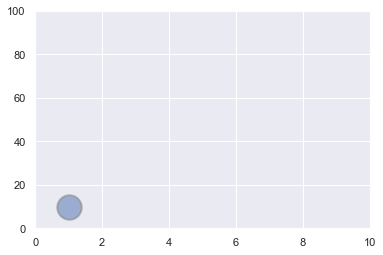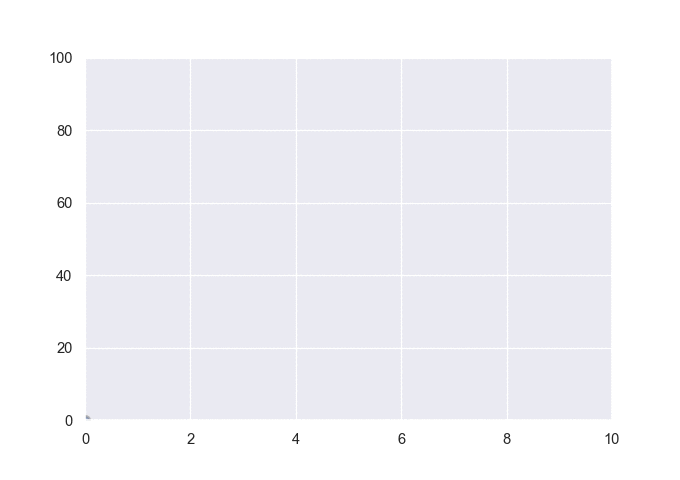This post explains how to build a very basic animated chart with `Python`. A loop is used to output several simple scatterplots at `png` format. A tool called `image magick` is then used to concatenate those images in a `gif`.

## 📍 Basic scatterplot

Let's start by building a very basic scatterplot. This is extensively described in the scatterplot section of the gallery, so let's go straight to the point:

``````# libraries
import matplotlib.pyplot as plt
import seaborn as sns

# grey background
sns.set(style="darkgrid")

# Scatterplot with 1 data point
plt.scatter(1, 10, s=600, alpha=0.5, edgecolors="grey", linewidth=2)
plt.xlim(0, 10)
plt.ylim(0, 100)
plt.show();

``````## 🔁 Loop

That was easy. Now let's build a loop that will produce this kind of chart at `.png` format at each iteration. For each step of the loop, the circle will be slightly moved toward the top right side of the figure:

``````# image resolution
dpi=96

# For each year:
for i in range(0,10):

# initialize a figure
fig = plt.figure(figsize=(680/dpi, 480/dpi), dpi=dpi)

# Build the scatterplot
plt.scatter(i, i*i, s=40+i*600, alpha=0.5, edgecolors="grey", linewidth=2)
plt.xlim(0, 10)
plt.ylim(0, 100)

# Save it & close the figure
filename='/Users/yan.holtz/Desktop/Scatter_step'+str(i)+'.png'
plt.savefig(fname=filename, dpi=96)
plt.gca()
plt.close(fig)``````

Now, you should have a set of 12 images in the specified folder (`/Users/yan.holtz/Desktop/` for me). Image magick is a command line tool that allows to concatenate those images in a `gif` file.

## ✨ Build a `Gif`

Install ImageMagick with this line of bash: `brew install imagemagick`

If you don't have `brew` installed, visit the image magick homepage for explanations.

Once the tool is installed, you can concatenate the 12 images using the following command:

``````# Bash
# convert -delay 80 Scatter*.png animated_scatter.gif

``````

Note: the above line of code is written in `bash`, not in `python`. You have to execute it in a terminal, not in your python environment.

And here is the final result## Contact & Edit

👋 This document is a work by Yan Holtz. You can contribute on github, send me a feedback on twitter or subscribe to the newsletter to know when new examples are published! 🔥